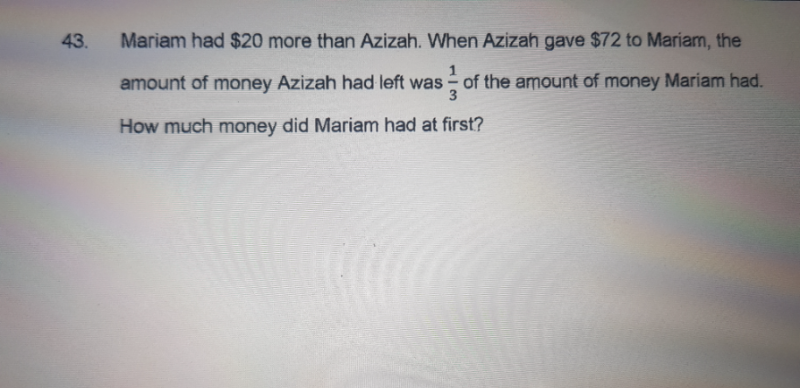# QuestionSubstituting
M – (1/3)M – 96 = 20
(2/3)M = 20 + 96 = 116
M = (3/2)116 = \$175 #

0 Replies 0 Likes

Thanks sushi88.  I thought I might supplement your answer with one based on equations 🙂

M – A = 20 …(1)

A – 72 =(1/3)(M + 72)
A = (1/3)(M + 72) + 72
A = (1/3)M + 96 …(2)

Substituting (2) into (1):
M – (1/3)M – 96 = 20
(2/3)M = 20 + 96 = 116
M = (3/2)116 = \$174 #

This question is slightly poorly worded by “1/3 the amount of money Mariam had” as it makes the reader wonder if it was Mariam’s original sum of money or it is after Mariam had received the money from Azizah.  I think if you do the question by models as you did, you may not see the problem, but the second equation could have been A – 72 = (1/3)M